Chargement...

# 7) Did the thermal resistance of the atmosphere increase? 7) Did the thermal resistance of the atmosphere increase?

7) Did the thermal resistance of the atmosphere increase?

Although the temperature at the surface level of the earth is + 15 [°C] (+59 [°F]), when observed from space the temperature of the planet is -19 [°C] (+2.2 [°F]) (see the folder Global Warming Part I).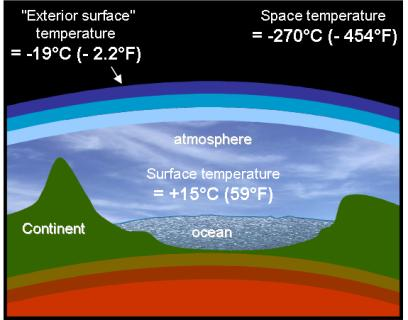The temperature at the surface level of the earth remains at +15 [°C] (+59 [°F]) due to the fact that some solar rays are neither immediately reflected towards space nor immediately absorbed by the atmosphere.
A large proportion of these rays penetrates through the atmosphere and reaches the ground. As a result the ground warms up and in return emits infrared rays.

The atmosphere captures 87% of these infrared rays coming from the ground, which allows the planet to keep its temperature around +15 [°C] (+59 [°F]) around the surface level while having a temperature of -19 [°C] (+2.2 [°F]) at 5,000 [m] altitude (according to measurements obtained with weather balloons).

In addition, the -19 [°C] (+2.2 [°F]) temperature is the one that allows the planet to discharge towards space the heat it receives from the sun and from the center of the earth as radiation.

As we have seen in the previous paragraphs, the variation or non variation of the temperature for which the planet is observed from space specifies the interpretation of Global Warming.

• If this temperature increased as much as the temperature at the surface level, the increase in thermal flux transmitted towards space is actually 60 times greater than our energy production. This could only be caused by a variation in solar activity, or a worse hypothesis is that it is due to an increase in the value of the absorption coefficient of our atmosphere.
• If this temperature did not vary, the hypothesis of a variation of solar activity or of the absorption coefficient of the atmosphere could not be plausible, because of the non increase in thermal flux emitted towards space. The temperature increase at the surface level could then be due to our human activity because of an increase in the thermal resistance of the atmosphere.

Because the variation of the rays emitted towards space to be detected is small, it is unlikely an analysis could have been performed based on the measures obtained by spatial probes over the past ten years.

For lack of better data, we are going to study the evolution of temperatures at 1,000 [m] and 4,400 [m] altitude measured by weather balloons between 1999 and 2008.

It is not obvious that the air temperature at around 5,000 [m] perfectly represents the temperature of the rays emitted by the planet. Therefore, part of the infrared rays emitted towards space (around 17%) comes directly from the surface of the earth.
However, the fact that this altitude corresponds closely to the equal sharing of superior and inferior layers of the atmosphere as far as their mass is concerned allows us to consider a certain correlation.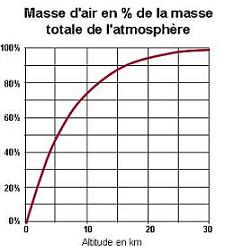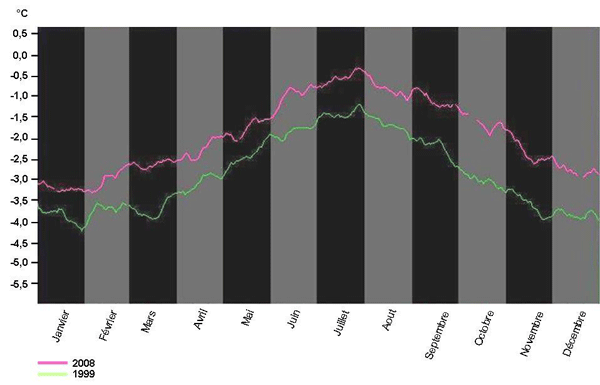Average temperature at 1,000 meters altitude in 1999 and 2008  in [°C]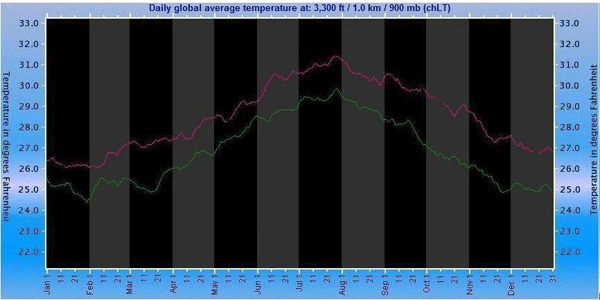Average temperature at 1,000 meters altitude in 1999 and 2008  in [°F]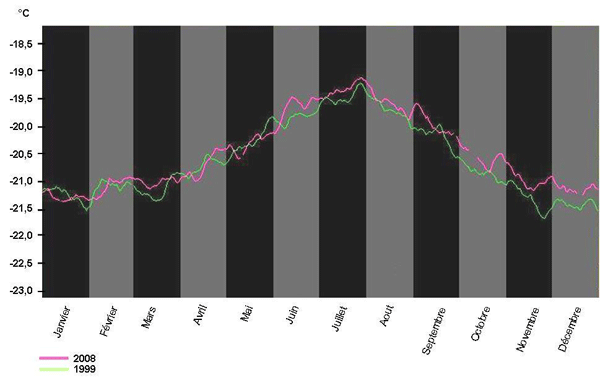Average temperature at 4,400 meters altitude in 1999 and 2008  in [°C]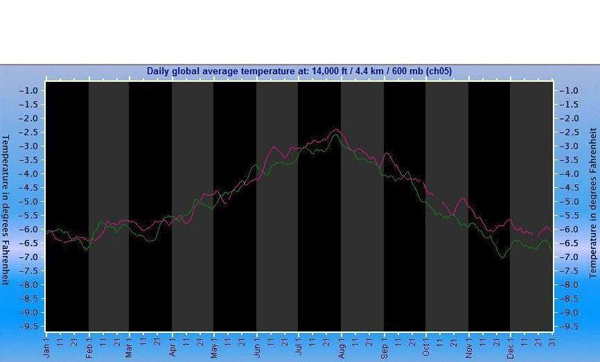Average temperature at 4,400 meters altitude in 1999 and 2008  in [°F]

Source AMSU-A Temperatures

## Question

If we compare the temperatures measured at 1,000 [m] and 4,400 [m], does the thermal resistance of the atmosphere seem to have increased between 1999 and 2008?

At 1,000 [m] altitude, we notice an observable temperature increase between 1999 and 2008.

In the mean time we notice that at 4,400 [m] altitude the temperature remained almost completely invariant.

As a consequence, the temperature difference between these two altitudes increased.

We denote R the thermal resistance of the atmosphere.

 In the construction domain, thermal resistance indicates in [m²] the necessary surface to transmit a power of 1 [W] through an inner wall, for 1 [°C] (1°F) temperature difference between the two sides of the surface. It is expressed in [m² °C/W].

We have R = ΔT / Flux (see the folder Global Warming Part I)

We can therefore write:

ΔT = R x Flux

The increase in ΔT could be due to an increase in the thermal resistance of the atmosphere or to that of the transmitted thermal flux.

If we admit that the temperature at 4,400 [m] is (at least partially) representative of the temperature of the planet observed from space, its small variation indicates a certain stability of the transmitted flux.

In this case it would therefore be the thermal resistance of the atmosphere that would have increased.

This would reinforce the hypothesis of a temperature increase which would be at least partially of anthropogenic origin (due to our activity), because if the temperature increase was essentially due to a variation in solar activity, it would have induced an increase in the thermal flux towards space, which appears to be invalidated by the stability of the temperature at 4,400 [m].